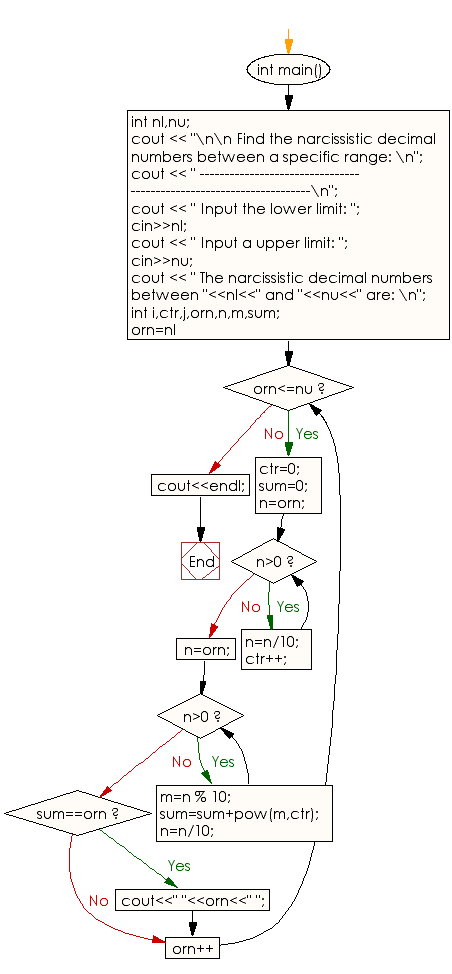﻿ C++ : Find Narcissistic decimal numbers within a range

# C++ Exercises: Find Narcissistic decimal numbers within a specific range

## C++ Numbers: Exercise-37 with Solution

Write a C++ program to find Narcissistic decimal numbers within a specific range.

Sample Solution:

C++ Code :

``````#include <iostream>
#include <cmath>
using namespace std;
int main()
{
int nl,nu;
cout << "\n\n Find the Narcissistic decimal numbers between a specific range: \n";
cout << " --------------------------------------------------------------------\n";
cout << " Input the lower limit: ";
cin>>nl;
cout << " Input a upper limit: ";
cin>>nu;
cout << " The Narcissistic decimal numbers between "<<nl<<" and "<<nu<<" are: \n";
int i,ctr,j,orn,n,m,sum;
for(orn=nl;orn<=nu;orn++)
{
ctr=0;
sum=0;
n=orn;
while(n>0)
{
n=n/10;
ctr++;
}
n=orn;
while(n>0)
{
m=n % 10;
sum=sum+pow(m,ctr);
n=n/10;
}
if(sum==orn)
{
cout<<" "<<orn<<" ";
}
}
cout<<endl;
}
``````

Sample Output:

```Find the Narcissistic decimal numbers between a specific range:
--------------------------------------------------------------------
Input the lower limit: 25
Input a upper limit: 200
The Narcissistic decimal numbers between 25 and 200 are:
153
```

Flowchart:C++ Code Editor:

What is the difficulty level of this exercise?

﻿

## C++ Programming: Tips of the Day

How to use doxygen to create UML class diagrams from C++ source

Doxygen creates inheritance diagrams but I dont think it will create an entire class hierachy. It does allow you to use the GraphViz tool. If you use the Doxygen GUI frontend tool you will find the relevant options in Step2: -> Wizard tab -> Diagrams. The DOT relation options are under the Expert Tab.

Ref: https://bit.ly/3QUFLBY

We are closing our Disqus commenting system for some maintenanace issues. You may write to us at reach[at]yahoo[dot]com or visit us at Facebook